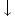# Day 105

## One-Dimension Multiple Charge Problems

So far we have seen that electrical forces can be calculated between two point charges.

What happens when there are more than two charges involved? The resulting force on a charged particle will be equal to the vector sum of all of the electrostatic forces acting on that particle. This is known as the Principle of Superposition.

Problem #1: Three charges lie on the x-axis.   A is -4.0 nC at x = 0.50 m, B is 5.0 nC at x=0, and C is 3.0 nC at x = 0.80 m. Find the magnitude and direction of the electrostatic force on the 5.0 nC charge, charge B.

Solution: The 5.0 nC charge is attracted to the 4 nC charge and repelled from the 3.0 nC charge. Therefore both forces will be directed to the left. In force notation, FBA <– (left) and FBC <– (left). (Remember FBA means the force that Charge B exerts on Charge A and FBC means the force that Charge B exerts on Charge C.)

Calculate the individual forces using Coulombs Law:

FBA = (9 x 109 Nm2/C2)(5.0 x 10-9 C)(-4.0 x 10-9 C)

(0.50 m)2

FBA = – 7.2 x10-7 N, left (negative indicates attraction, not magnitude)

FBC = (9 x 109 Nm2/C2)(5.0 x 10-9 C)(3.0 x 10-9 C)

(0.80 m)2

FBC = +2.1 x 10-7 N, left (positive indicates repulsion, not magnitude)

FB = FBA + FBC = 9.3 x 10-7 N, left

Problem #2: Using the same charges as Problem #1,

The 1mC charge is at the top of the triangle, the 2mC charge is at the left side of the base,

and the 3mC charge is on the right side of the base,

find the net force on Charge C.

FCA = (9 x 109 Nm2/C2)(3.0 x 10-9 C)(-4.0 x 10-9 C), LEFT (attracted)

(1.30 m)2

FCA = -6.4 x 10-8 N, left

FCB = (9 x 109 Nm2/C2)(3.0 x 10-9 C)(5.0 x 10-9 C), right (repelled)

(0.80 m)2

FCB = +2.1 x 10-7 N, right

(NOTICE: FBC = -FCB)

Since the two vector quantities are opposite in direction, we take the difference between their magnitudes (ignore the signs) and assign the direction of the greater individual magnitude.

FC = |FCA – FCB|

FC = 1.5 x 10-7 N, right

## Two-Dimension Multiple Charge Problems

Since electrostatic forces are vectors, when multiple charges are found on different axes, the resultant force is the vector sum of the individual forces. Trigonometry must be used, just as in any other vector problem.

Problem #1: Three charges are located at the vertices of an equilateral triangle that has sides of length 15 cm. Find the net force on the 2.0 μC charge.

Solution:

Draw a free body diagram showing the forces acting on the 2.0 μC charge.

The 2.0 μC charge is repelled from the 1.0 μC charge and attracted to the -3.0 μC charge.Each vector must be separated in x and y components:

F(2,1) x = (9 x 109 Nm2/C2)(2.0 x 10-6 C)(1.0 x 10-6 C) cos 60

(0.15 m)2

= 5.0 x 10-1 N,F(2,1) y = (9 x 109 Nm2/C2)(2.0 x 10-6 C)(1.0 x 10-6 C) sin 60

(0.15 m)2

= 6.9 x 10-1 N,F(2,-3) x = (9 x 109 Nm2/C2)(2.0 x 10-6 C)(-3.0 x 10-6 C) cos 0

(0.15 m)2

= 2.4 NF(2,-3) y = (9 x 109 Nm2/C2)(2.0 x 10-6 C)(-3.0 x 10-6 C) sin 0

(0.15 m)2

= 0 N

F2 x = | F2,1 – F2, -3 |

F2x = 1.9 N,F2y = 6.9 x 10-1 N + 0 = 6.9 x 10-1 NF2 = √ (1.9 N)2 + (0.69 N)2 =

F2 = 2.0 N

Θ = tan-1 Fy/Fx

Θ = tan-1 (0.69N/1.9 N)

Θ = 20.0o

Since the resultant vector is in the 4th quadrant, the angle with respect to the x-axis, is

360o – 20o = 340o

### Try the following problems:

1) How many electrons are contained in 1 C of charge? What is the mass of the electrons in 1 C of charge?

2) If two equal charges, each of 1 C, were separated in air by a distance of 1 km, what would be the force between them?

3) Determine the force between two free electrons spaced 1 angstrom (10-10 m) apart.

4) What is the force of repulsion between two argon nuclei that are separated by 1 nm? The charge on an argon nucleus is +18e.

5) Two equally charged balls are 3 cm apart in air and repel each other with a force of 40 μN. Compute the charge on each ball.

6) Three point charges are placed at the following points on the x-axis: +2 μC at x=0, =3 μC at x=40 cm, -5 μC at x=120 cm. Find the force on (A) the =3 μC charge and (B) the -5 μC charge.

7) Four equal point charges of +3μ C are placed at the four corners of a square that is 40 cm on a side. Find the force on any one of the charges.

8) Four equal magnitude point charges (3 μC) are placed at the corners of a square that is 40 cm on a side. Two, diagonally opposite each other, are positive, and the other two are negative. Find the force on either negative charge.

9) Charges of +2, +3, and-8 μC are placed at the vertices of an equilateral triangle of side 10. cm. Calculate the magnitude of the force acting on the -8 μC charge due to the other two charges.

10) One charge (+5 μC) is placed at x=0, and a second charge (+7 μC) at x=100 cm. Where can a third charge be placed so as to experience zero net force due to the other two?

11) Two identical tiny metal balls carry charges of +3 nC and -12 nC. They are 3 cm apart. (A) Compute the force of attraction. (B) The balls are now touched together and then separated to 3 cm. Describe the forces on them now.

(source)